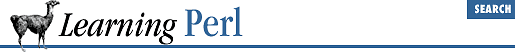home | O'Reilly's CD bookshelfs | FreeBSD | Linux | Cisco | Cisco Exam

### 15.2 Extracting and Replacing a Substring

Pulling out a piece of a string can be done with careful application of regular expressions, but if the piece is always at a known character position, this is inefficient. Instead, you should use ``` substr``` . This function takes three arguments: a string value, a start position (measured like it was measured for ``` index``` ), and a length, like so:

```\$s = substr(```

\$string
```
,```

\$start
```
,```

\$length
```
);```

The start position works like ``` index``` : the first character is zero, the second character is one, and so on. The length is the number of characters to grab at that point: a length of zero means no characters, one means get the first character, two means two characters, and so on. (It stops at the end of the string, so if you ask for too many, it's no problem.) It looks like this:

```\$hello = "hello, world!";
\$grab  = substr(\$hello, 3, 2);   # \$grab gets "lo"
\$grab  = substr(\$hello, 7, 100); # 7 to end, or "world!"```

You could even create a " ten to the power of " operator for small integer powers, as in:

`\$big = substr("10000000000",0,\$power+1); # 10 ** \$power`

If the count of characters is zero, an empty string is returned. If either the starting position or ending position is less than zero, the position is counted that many characters from the end of the string. So ``` -1``` for a start position and ``` 1``` (or more) for the length gives you the last character. Similarly, ``` -2``` for a start position starts with the second-to-last character like this:

```\$stuff = substr("a very long string",-3,3); # last three chars
\$stuff = substr("a very long string",-3,1); # the letter "i"```

If the starting position is before the beginning of the string (like a huge negative number bigger than the length of the string), the beginning of the string is the start position (as if you had used ``` 0``` for a starting position). If the start position is a huge positive number, the empty string is always returned. In other words, it probably does what you expect it to do, as long as you expect it to always return something other than an error.

Omitting the length argument is the same as if you had included a huge number for that argument - grabbing everything from the selected position to the end of the string.[ 1 ]

 Very old Perl versions did not allow the third argument to be omitted, leading to the use of a huge number for that argument by pioneer Perl programmers. You may come across this in your Perl archeological expeditions.

If the first argument to ``` substr``` is a scalar variable (in other words, it could appear on the left side of an assignment operator), then the ``` substr``` itself can appear on the left side of an assignment operator. This may look strange if you come from a C background, but if you've ever played with some dialects of BASIC, it's quite normal.

What gets changed as the result of such an assignment is the part of the string that would have been returned had the ``` substr``` been used on the right-hand side of the expression instead. In other words, ``` substr(\$var,3,2)``` returns the fourth and fifth characters (starting at ``` 3``` , for a count of ``` 2``` ), so assigning to that changes those two characters for ``` \$var``` like so:

```\$hw = "hello world!";
substr(\$hw, 0, 5) = "howdy"; # \$hw is now "howdy world!"```

The length of the replacement text (what gets assigned into the ``` substr``` ) doesn't have to be the same as the text it is replacing, as it was in this example. The string will automatically grow or shrink as necessary to accommodate the text. Here's an example where the string gets shorter:

`substr(\$hw, 0, 5) = "hi"; # \$hw is now "hi world!"`

and here's one that makes it longer:

`substr(\$hw, -6, 5) = "nationwide news"; # replaces "world"`

The shrinking and growing are fairly efficient, so don't worry about using them arbitrarily, although it is faster to replace a string with a string of equal length.15.1 Finding a Substring15.3 Formatting Data with sprintf( )